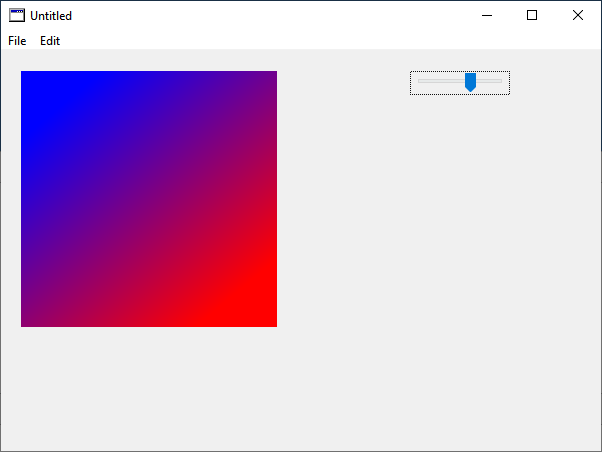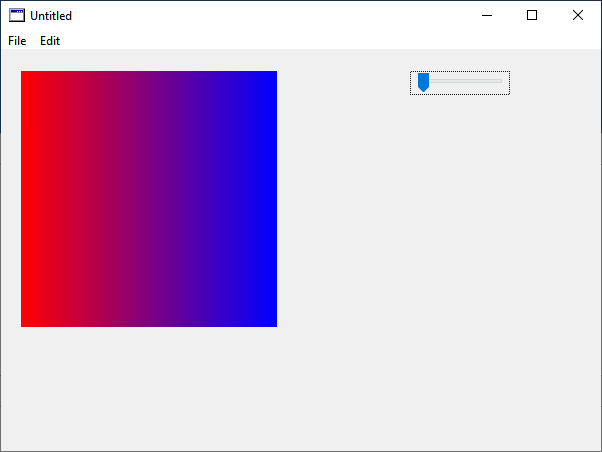# How to calculate correct endpoint

Hi everyone,

once again a question to the mathematicians among usI have a class `MyRectangle` with the properties `Bounds As Xojo.Rect` and `Angle As Double` (Radians).

Now I want to draw this object on the Canvas with a `LinearGradientBrush`. How can I calculate the correct endpoint so that the gradient within the rect has the correct angle?

``````Var obj As New MyRectangle

obj.Angle = 0.785398 ' 45 degree
obj.Bounds = New Xojo.Rect(0, 0, 200, 200)

' Drawing

grad.EndPoint = // how to calculate?

g.FillRectangle(obj.Bounds.Left, obj.Bounds.Top, obj.Bounds.Width, obj.Bounds.Height)
``````

just think the color / Gradient that will be drawn is between two fingers.
you can draw from left top to right bottom diagonal or you can also draw from top to bottom with the same left value.

Yes Markus, I know, I am interested in how to calculate the correct Start/EndPoint for LinearGradientBrush from a given rectangle and angle.

Given the start point A, the end point B will be start + length * angle.

A = (rect.left, rect.top)
length = rect.width (horizontal) or rect.height (vertical)
B = Ax + length * Cos(angleRadians), Ay + length * Sin(angleRadians)

Since in Xojo the coordinate system has the origin in the top left, you’d have to invert the Y-axis: By = Ay - length * Sin(angleRadians)

Of course you can also define arbitrary values for A and length to change the orientation of the gradient.

1 Like

this cos and sin gave you a direction vector which is between -1 and 1
that is just stretched by multiplied with a length.
you can add x and y as start point and the result is the end of track.

Here you can see what I did in a `Canvas.Paint`-Event:

``````Static obj As MyRectangle
Static aX As Double
Static aY As Double

Const kPi = 3.14159265359

If obj Is Nil Then

obj = New MyRectangle
obj.Bounds = New Rect(20, 20, 200, 200)

aX = obj.Bounds.Left
aY = obj.Bounds.Top

End If

' Drawing
obj.Angle = Slider1.Value * kPi / 180

grad.EndPoint = New Point(aX + obj.Bounds.Width * Cos(obj.Angle), aY + obj.Bounds.Height * Sin(obj.Angle))

g.FillRectangle(obj.Bounds.Left, obj.Bounds.Top, obj.Bounds.Width, obj.Bounds.Height)
``````

Here’s what I’m looking for:

Looks also like StartPoint becomes incorrect when changing the angle.

I have never used gradientbrush, but from what I see in your video I would say for angles above 90º it fails because your starting point needs to adapt to the direction. Try moving it to the top right corner for angles between 90 and 180 degrees, bottom right for angles between 180 and 270, and bottom left for angles between 270 and 360.

If you try, please let us know what you get, thanks.

EDIT: I would try myself but I don’t have the latest version of Xojo installed.

Julen

Ah, well, I understood you wanted a rotated rectangle with a gradient, but I see you actually want a rectangle with a rotated gradient. That’s a bit different. Rotating the gradient requires a live calculation of the start point as well. In this case, you’ll use the centre of the rectangle and calculate the two points as the endpoints of the circumscribed circle. See the comments in the code, they will make it clearer.

``````Static obj As MyRectangle

Const kPi = 3.14159265359

If obj Is Nil Then
// Init rectangle
obj = New MyRectangle
obj.Bounds = New Rect(20, 20, 200, 200)

End If

// Define radius as the diagonal of the rectangle, i.e. define the circumscribed circle
// This way the gradient will fill the entire shape
var radius As Double = Sqrt(obj.bounds.Width*obj.bounds.Width + obj.bounds.Height*obj.bounds.Height) / 2

// Define angle
var angle As Double = Slider1.Value * kPi / 180

// Precalculate offsets
var deltaX As Double = radius * Cos(angle)
var deltaY As Double = radius * Sin(angle)

// Store the centre coordinates
var Cx As Double = obj.bounds.Center.X
var Cy As Double = obj.bounds.Center.Y

// Define start & end points of the gradient
grad.StartPoint = New Point(Cx - deltaX, Cy - deltaY)
grad.EndPoint = New Point(Cx + deltaX, Cy + deltaY)

// Draw
g.FillRectangle(obj.Bounds.Left, obj.Bounds.Top, obj.Bounds.Width, obj.Bounds.Height)``````
2 Likes

Thank you so much @Andrea_Suraci for this explanation, this works like charm. You can be sure that more mathematical questions will comeThe only thing I notice now is that the colors no longer start at the mentioned positions within the rectangle. Do you have to adjust their percentages to the angle?

OK, the calculation is already close to what I need.

Compare the two screenshots below: They show the gradient filled rectangle in Apple Pages and the Xojo generated one. The one generated by Xojo shows an offset of the colors. The red/blue color does not start/end exactly. How can you match this to the look of Apple Pages?

230°

i guess because the radius variable use half diagonal.
seems your start and endpoint is outside.

Yes, somehow you now have to adjust the original GradientStop percent values. Complicated! Because the values for gradients with more than two colors must always remain in the correct % ratio.

check this, i use a canvas paint event

``````Sub Paint(g As Graphics, areas() As REALbasic.Rect) Handles Paint

Const rad As Double = 0.0174533

Var radius As Double = Min(Me.Width,Me.Height) / 2.0

Var angle As Double = Slider1.Value * rad  'Slider1 in degree 0-360

Var deltaX As Double = radius * Cos(angle)
Var deltaY As Double = radius * Sin(angle)

Var Cx As Double = Me.Width / 2.0
Var Cy As Double = Me.Height / 2.0

grad.StartPoint = New Point(Cx - deltaX, Cy - deltaY)
grad.EndPoint = New Point(Cx + deltaX, Cy + deltaY)

g.FillRectangle(0,0,Me.Width,Me.Height)
End Sub
``````1 Like

Odd, in my tests using Min(width, height) did not fill the extra space with the solid color. I’m working on a Mac, so perhaps that’s a difference between CoreGraphics and Direct2D.

2 Likes

Thanks @MarkusR, that’s much better. I agree to @Andrea_Suraci, that there are differences between the drawing on Windows and macOS. To prevent us from this, I’ve added two lines of code, to take care of it on macOS.

``````Const rad As Double = 0.0174533

Var radius As Double = Min(Me.Width, Me.Height) / 2.0
Var angle As Double = Slider1.Value * rad  ' Slider1 in degree 0-360

Var deltaX As Double = radius * Cos(angle)
Var deltaY As Double = radius * Sin(angle)

Var Cx As Double = Me.Width / 2.0
Var Cy As Double = Me.Height / 2.0

grad.StartPoint = New Point(Cx - deltaX, Cy - deltaY)
grad.EndPoint = New Point(Cx + deltaX, Cy + deltaY)

' Fix for macOS
g.FillRectangle(0, 0, Me.Width, Me.Height)

g.FillRectangle(0, 0, Me.Width, Me.Height)
``````

1 Like

Hi @Andrea_Suraci, @MarkusR, @Julen_I and all other,

I come back to this issue. The above solutions are good for rectangles with the same edge length on all sides (squares). However, the positions of the ColorStops (percentages) are not adjusted correctly when it is a rectangle.

Here you can see a rectangle filled with a gradient. The two black points are the start and end points of the gradient.

The `Paint`-Event code for this looks like this:

``````Const rad As Double = 0.0174533

Var radius As Double = Min(Me.Width, Me.Height) / 2.0
Var angle As Double = Slider1.Value * rad ' Slider1 in degree 0-360

Var deltaX As Double = radius * Cos(angle)
Var deltaY As Double = radius * Sin(angle)

Var Cx As Double = Me.Width / 2.0
Var Cy As Double = Me.Height / 2.0

grad.StartPoint = New Point(Cx - deltaX, Cy - deltaY)
grad.EndPoint = New Point(Cx + deltaX, Cy + deltaY)

g.FillRectangle(0, 0, Me.Width, Me.Height)

' Start-/EndPoint
g.Brush = Nil
``````

And here’s a video from Norman that he posted on his blog. However, due to copyright issues, he cannot publish the code that ensures that the ColorStops are positioned correctly.

Does anyone have an idea, an approach, how to modify the code to always get a uniformly filled rectangle?

Thanks

I guess the idea should be to rotate square rectangle (used to start/stop gradient points) where the length of the side of the square is equal to a diagonal of the canvas-drawn rectangle and where centres of both square (the gradient details are based on) and rectangle are of the same coordinates.

in your example code i would longer the radius and then limit the position to the rectangle.

Hi @Pawel_Soltysinski, morning @MarkusR, thanks for your suggestion. Can you show a bit of code please?

ok, when I get back home I will try to develop the solution.

1 Like